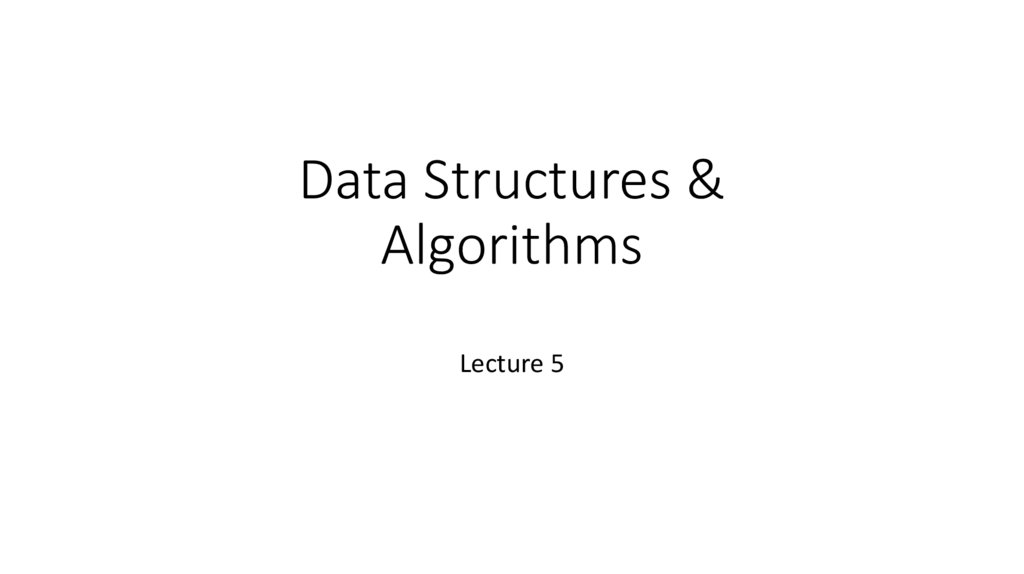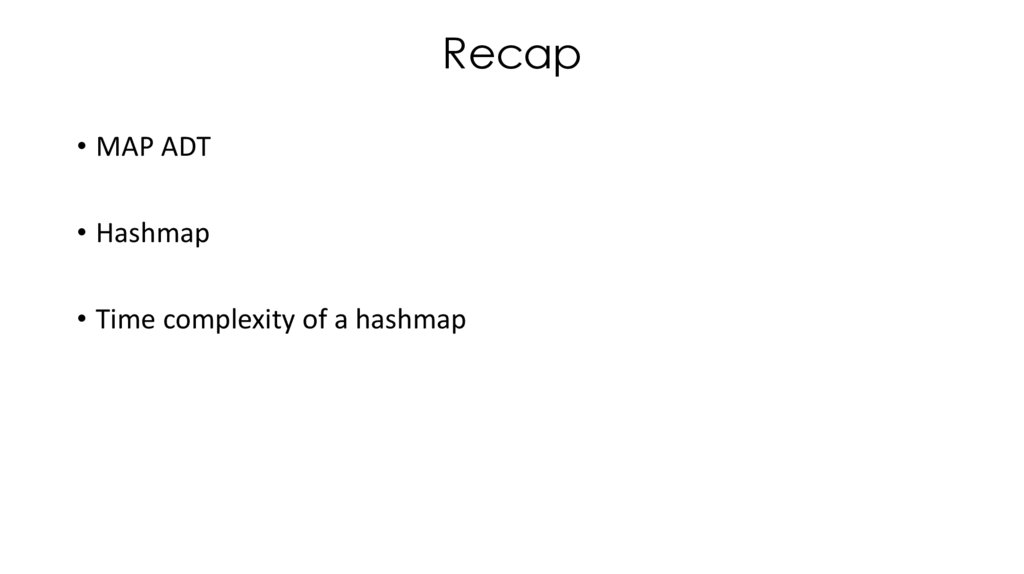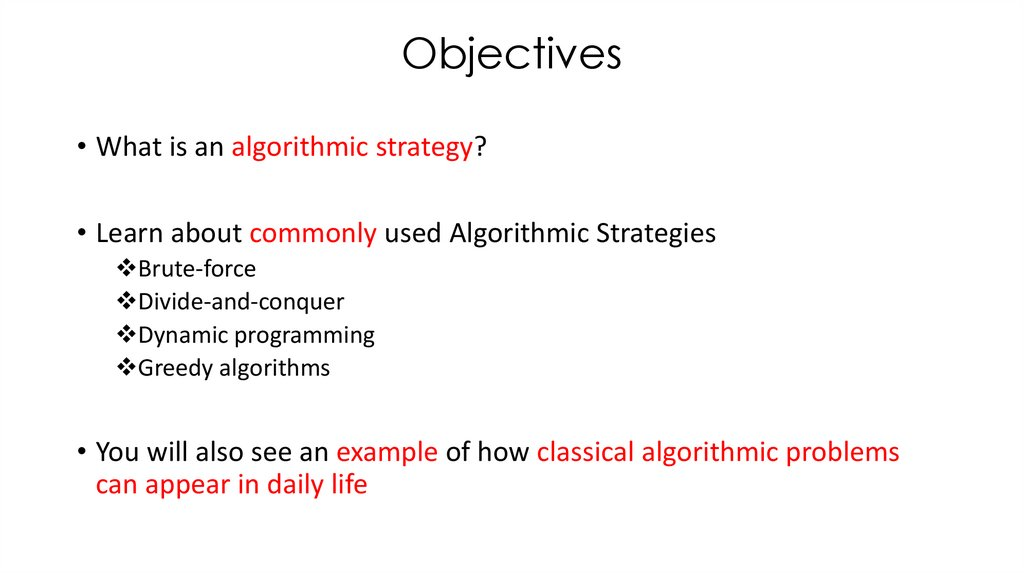# Algorithmic strategies

## 1. Data Structures & Algorithms

Data Structures &
Algorithms
Lecture 5

## 2.

Recap
• Hashmap
• Time complexity of a hashmap

## 3.

Objectives
• What is an algorithmic strategy?
• Learn about commonly used Algorithmic Strategies
Brute-force
Divide-and-conquer
Dynamic programming
Greedy algorithms
• You will also see an example of how classical algorithmic problems
can appear in daily life

## 4. Algorithm Classification

• Based on problem domain
• Based on algorithmic strategy

## 5.

Algorithmic Strategies
• Approach to solving a problem
• Algorithms that use a similar problem solving approach can be
grouped together
• Classification scheme for algorithms

Brute Force

## 7.

Brute Force
• Straightforward approach to solving a problem based on the simple
formulation of the problem
• Often, does not require deep analysis of the problem
• Perhaps the easiest approach to apply and is useful for solving
problems of small-size

## 8.

Brute Force
• May results in naïve solutions with poor performance
• Examples
Computing